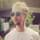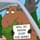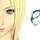It seems that you're using an outdated browser. Some things may not work as they should (or don't work at all).
We suggest you upgrade newer and better browser like: Chrome, Firefox, Internet Explorer or Opera

×
Time for yet another local prices update.

OLD
5.99 USD = 5.29 eur = 3.89 GBP = 8.49 AUD
9.99 USD = 8.79eur = 6.49 GBP = 14.09 AUD

NEW:
5.99 USD = 5.39 EUR = 3.99 GBP = 8.59 AUD
9.99 USD = 8.99 EUR = 6.59 GBP = 14.39 AUD
- Knock knock
- Who's there?
- Currency fluctuations!

In short, the prices have updated once more. Here are the main GOG price points for games and their local equivalents:

\$5.99 = €5.49 = £3.89 = A\$8.39
\$9.99 = €9.09 = £6.49 = A\$14.09
\$14.99 = €13.69 = £9.79 = A\$21.09

Remember that if your local price is greater than the game's value in USD, you're gonna get the difference returned straight to your GOG.com account thanks to our Fair Price Package. :)
Post edited November 04, 2015 by Ciris
Currencies sure aren't calming down - the prices have been changed once more.

\$ ------ EUR ---- GBP ---- AUD
5,99 --- 5,69 --- 3,99 --- 8,49
9,99 --- 9,39 --- 6,59 --- 14,09
14,99 -- 14,09 -- 9,89 --- 21,09
Post edited November 18, 2015 by Ciris
And things had to change yet again. Those currency exchange rates sure are crazy!

Here are the up-to-date price points:

\$ ------- EUR ----- GBP ------ AUD
5,99 ---- 5,69 ----- 3,99 ----- 8,39
9,99 ---- 9,49 ----- 6,69 ---- 13,89
14,99 -- 14,19 ----- 9,99 ---- 20,89
Currencies! Global market! Economy! KABOOM!

We've had to readjust our prices once more - here are the main changes:

OLD:

\$ 5.99 = € 5.69 = £ 3.99 = A\$ 8.39 = ₽ 399
\$ 9.99 = € 9.49 = £ 6.69 = A\$ 13.89 = ₽ 669

NEW:

\$ 5.99 = € 5.49 = £ 3.99 = A\$ 8.29 = ₽ 399
\$ 9.99 = € 9.09 = £ 6.69 = A\$ 13.89 = ₽ 669
Post edited December 16, 2015 by Ciris
Hey everyone, we've got yet another change of price tags to keep up with this dynamic and complicated world of finance:

OLD:
5.99\$ = 5.49 EUR = 3.99 GBP = 8.29 AUD = 419 RUB
9.99\$ = 9.09 EUR = 6.69 GBP = 13.89 AUD = 709 RUB

NEW:
5.99\$ = 5.49 EUR = 4.19 GBP = 8.59 AUD = 459 RUB
9.99\$ = 9.19 EUR = 6.89 GBP = 14.39 AUD = 759 RUBGeorgeTheShovel: Hey everyone, we've got yet another change of price tags to keep up with this dynamic and complicated world of finance:

OLD:
5.99\$ = 5.49 EUR = 3.99 GBP = 8.29 AUD = 419 RUB
9.99\$ = 9.09 EUR = 6.69 GBP = 13.89 AUD = 709 RUB

NEW:
5.99\$ = 5.49 EUR = 4.19 GBP = 8.59 AUD = 459 RUB
9.99\$ = 9.19 EUR = 6.89 GBP = 14.39 AUD = 759 RUBHunchBluntley: New to the GOG team? (Love the nickname, by the way! =D )
Kinda: been here for a month now) I'm here to look after our Russian-speaking users, mostly, but not exclusively as you can see!

Thx, btw! In return, love your avatar :D
My turn! Have some currency updates, friends:

OLD
5.99\$ = 5.49 EUR = 4.19 GBP = 8.59 AUD = 459 RUB
9.99\$ = 9.19 EUR = 6.89 GBP = 14.39 AUD = 759 RUB

NEW
5.99\$ = 5.39 EUR = 4.19 GBP = 8.39 AUD = 469 RUB
9.99\$ = 8.99 EUR = 6.89 GBP = 13.99 AUD = 779 RUB
Changes, changes, changes!

OLD:
5.99\$ = 5.39 EUR = 4.19 GBP = 8.39 AUD = 469 RUB
9.99\$ = 8.99 EUR = 6.89 GBP = 13.99 AUD = 779 RUB

NEW:
5.99\$ = 5.39 EUR = 4.19 GBP = 7.99 AUD = 419 RUB
9.99\$ = 8.99 EUR = 6.99 GBP = 13.19 AUD = 699 RUB
There's an update indeed! Here are the official values (with a bunch of new currencies!):

5.99 USD = 5.19 EUR = 4.09 GBP = 7.89 AUD = 399 RUB = 22.79 PLN = 48 SEK = 48 NOK = 39 DKK = 5.79 CHF = 7.59 CAD

9.99 USD = 8.69 EUR = 6.89 GBP = 13.19 AUD = 669 RUB = 37.99 PLN = 80 SEK = 80 NOK = 64 DKK = 9.59 CHF = 12.59 CAD

14.99 USD = 12.99 EUR = 10.29 GBP = 19.79 AUD = 999 RUB = 56.99 PLN = 119 SEK = 120 NOK = 97 DKK = 14.29 CHF = 18.89 CAD
A new day, a new set of currency conversions. Grab 'em while they're hot!

5,99USD = 5,29EUR = 4,19GBP = 8,19AUD = 399 RUB = 22,99PLN = 49SEK = 49NOK = 39DKK = 5,89CHF = 7,79 CAD

9,99USD = 8,79EUR = 6,99 GBP = 13.59 AUD = 659RUB = 38,49 PLN = 81SEK = 82NOK = 65 DKK = 9,79 CHF = 12,99 CAD

14,99USD = 13,29EUR = 10,39GBP = 20,39AUD = 989RUB = 57,79 PLN = 122SEK = 123NOK = 98DKK = 14,69CHF = 19,39 CAD
Post edited June 08, 2016 by KonradKonrad: 5,99USD = ... = 7,79CHFmrkgnao: Should be CAD, I believe.
Fixed! Thank you. :)
You guys noticed already, but there was indeed an update! Here are the new rates:

5,99USD = 5,49EUR = 4,49GBP = 8,09AUD = 399 RUB = 24,39PLN = 51,10SEK = 51,20NOK = 40,40DKK = 5,89CHF = 7,89CAD

9,99USD = 9,09EUR = 7,59 GBP = 13,59 AUD = 659RUB = 40,29PLN = 85,30SEK = 85,40NOK = 67,40DKK = 9,79CHF = 13,09CAD

14,99USD = 13,59EUR = 11,29GBP = 20,29AUD = 979RUB = 60,39PLN = 128SEK = 128,20NOK = 101,10DKK = 14,69CHF = 19,59 CAD
More local! More prices! More update!

5.99 USD = 5.39 EUR = 4,69 GBP = 7,89 AUD = 389 RUB = 22.99 PLN = 50.50 SEK = 51,20 NOK = 39,90 DKK = 5.89 CHF = 7.49 CAD

9.99 USD = 8.99 EUR = 7.79 GBP = 13.09 AUD = 649 RUB = 38.29 PLN = 84.3 SEK = 81.8 NOK = 66.5 DKK = 9.79 CHF = 12.39 CAD

14.99 USD = 13.49 EUR = 11.59 GBP = 19.59 AUD = 979 RUB = 57.39 PLN = 126.5 SEK = 122.7 NOK = 99.8 DKK = 14.69 CHF = 18.59 CAD
Meanwhile, the world stage shifts ever so slightly.

5.99 USD = 5.49 EUR = 4.99 GBP = 7.89 AUD = 379 RUB = 23.69 PLN = 54.00 SEK = 49.30 NOK = 40.60 DKK = 5.89 CHF = 8.09 CAD = 19.39 BRL

9.99 USD = 9.09 EUR = 8.19 GBP = 13.09 AUD = 639 RUB = 39.09 PLN = 89.80 SEK = 82.30 NOK = 67.50 DKK = 9.89 CHF = 13.39 CAD = 32.39 BRL

14.99 USD = 13.69 EUR = 12.29 GBP = 19.69 AUD = 949 RUB = 58.69 PLN = 134.80 SEK = 123.40 NOK = 101.30 DKK = 14.79 CHF = 20.09 CAD = 48.49 BRL